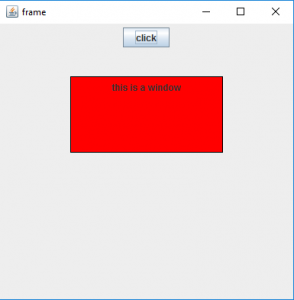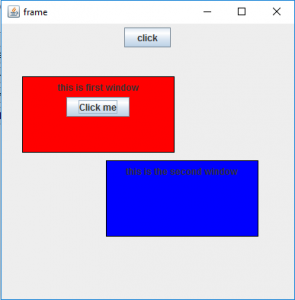GFG App
Open AppBrowser
Continue

# Java Swing | JWindow with examples

JWindow is a part of Java Swing and it can appear on any part of the users desktop. It is different from JFrame in the respect that JWindow does not have a title bar or window management buttons like minimize, maximize, and close, which JFrame has. JWindow can contain several components such as buttons and labels.
Constructor of the class are:

1. JWindow() : creates an empty Window without any specified owner
2. JWindow(Frame o) :creates an empty Window with a specified frame as its owner
3. JWindow(Frame o) : creates an empty Window with a specified frame as its owner
4. JWindow(Window o) : creates an empty Window with a specified window as its owner
5. JWindow(Window o, GraphicsConfiguration g) : creates an empty window with a specified window as its owner and specified graphics Configuration.
6. JWindow(GraphicsConfiguration g) :creates an empty window with a specified Graphics Configuration g.

Commonly used methods

1. setLayout(LayoutManager m) : sets the layout of the Window to specified layout manager
2. setContentPane(Container c) : sets the ContentPane property of the window
3. getContentPane() : get the container which is the ContentPane for this Window
5. isVisible(boolean b): sets the visibility of the Window, if value of the boolean is true then visible else invisible
6. update(Graphics g) : calls the paint(g) function
7. remove(Component c) : removes the component c
8. getGraphics() : returns the graphics context of the component.
9. getLayeredPane() : returns the layered pane for the window
10. setContentPane(Container c) :sets the content pane for the window
11. setLayeredPane(JLayeredPane l) : set the layered pane for the window
12. setRootPane(JRootPane r) : sets the rootPane for the window
13. setTransferHandler(TransferHandler n) : Sets the transferHandler property, which is a mechanism to support transfer of data into this component.
14. setRootPaneCheckingEnabled(boolean enabled) : Sets whether calls to add and setLayout are forwarded to the contentPane.
15. setRootPane(JRootPane root) :Sets the rootPane property of the window.
16. setGlassPane(Component glass) : Sets the glassPane property of the window.
17. repaint(long time, int x, int y, int width, int height): Repaints the specified rectangle of this component within time milliseconds.
18. remove(Component c): Removes the specified component from the window.
19. isRootPaneCheckingEnabled() : Returns whether calls to add and setLayout are forwarded to the contentPane or not .
20. getTransferHandler() : returns the transferHandler property.
21. getRootPane() : Returns the rootPane object for this window.
22. getGlassPane() : Returns the glassPane object for this window.
23. createRootPane() : Called by the constructor methods to create the default rootPane.
24. addImpl(Component co, Object c, int i) : Adds the specified child Component to the window.

The following programs will illustrate the use of JWindow

1. program to create a simple JWindow

 `// java Program to create a simple JWindow ` `import` `java.awt.event.*; ` `import` `java.awt.*; ` `import` `javax.swing.*; ` ` `  ` `  `class` `solveit ``extends` `JFrame ``implements` `ActionListener { ` ` `  `    ``// frame ` `    ``static` `JFrame f; ` ` `  `    ``// main class ` `    ``public` `static` `void` `main(String[] args) ` `    ``{ ` `        ``// create a new frame ` `        ``f = ``new` `JFrame(``"frame"``); ` ` `  `        ``// create a object ` `        ``solveit s = ``new` `solveit(); ` ` `  `        ``// create a panel ` `        ``JPanel p = ``new` `JPanel(); ` ` `  `        ``JButton b = ``new` `JButton(``"click"``); ` ` `  `        ``// add actionlistener to button ` `        ``b.addActionListener(s); ` ` `  `        ``// add button to panel ` `        ``p.add(b); ` ` `  `        ``f.add(p); ` ` `  `        ``// set the size of frame ` `        ``f.setSize(``400``, ``400``); ` ` `  `        ``f.show(); ` `    ``} ` ` `  `    ``// if button is pressed ` `    ``public` `void` `actionPerformed(ActionEvent e) ` `    ``{ ` `        ``String s = e.getActionCommand(); ` `        ``if` `(s.equals(``"click"``)) { ` `            ``// create a window ` `            ``JWindow w = ``new` `JWindow(f); ` ` `  `            ``// set panel ` `            ``JPanel p = ``new` `JPanel(); ` ` `  `            ``// create a label ` `            ``JLabel l = ``new` `JLabel(``"this is a window"``); ` ` `  `            ``// set border ` `            ``p.setBorder(BorderFactory.createLineBorder(Color.black)); ` ` `  `            ``p.add(l); ` `            ``w.add(p); ` ` `  `            ``// set background ` `            ``p.setBackground(Color.red); ` ` `  `            ``// setsize of window ` `            ``w.setSize(``200``, ``100``); ` ` `  `            ``// set visibility of window ` `            ``w.setVisible(``true``); ` ` `  `            ``// set location of window ` `            ``w.setLocation(``100``, ``100``); ` `        ``} ` `    ``} ` `} `

Output :1. program to create a multiple JWindow .( where one window is the owner of the other )

 `// java program to create a multiple  JWindow .( where one window is the owner of the other )< ` `import` `java.awt.event.*; ` `import` `java.awt.*; ` `import` `javax.swing.*; ` `class` `solveit ``extends` `JFrame ``implements` `ActionListener { ` ` `  `    ``// frame ` `    ``static` `JFrame f; ` ` `  `    ``// windows ` `    ``JWindow w, w1; ` ` `  `    ``// object of class ` `    ``static` `solveit s; ` ` `  `    ``// main class ` `    ``public` `static` `void` `main(String[] args) ` `    ``{ ` `        ``// create a new frame ` `        ``f = ``new` `JFrame(``"frame"``); ` ` `  `        ``// create a object ` `        ``s = ``new` `solveit(); ` ` `  `        ``// create a panel ` `        ``JPanel p = ``new` `JPanel(); ` ` `  `        ``JButton b = ``new` `JButton(``"click"``); ` ` `  `        ``// add actionlistener to button ` `        ``b.addActionListener(s); ` ` `  `        ``// add button to panel ` `        ``p.add(b); ` ` `  `        ``f.add(p); ` ` `  `        ``// set the size of frame ` `        ``f.setSize(``400``, ``400``); ` ` `  `        ``f.show(); ` `    ``} ` ` `  `    ``// if button is pressed ` `    ``public` `void` `actionPerformed(ActionEvent e) ` `    ``{ ` `        ``String s1 = e.getActionCommand(); ` `        ``if` `(s1.equals(``"click"``)) { ` `            ``// create a window ` `            ``w = ``new` `JWindow(f); ` ` `  `            ``// set panel ` `            ``JPanel p = ``new` `JPanel(); ` ` `  `            ``// create a label ` `            ``JLabel l = ``new` `JLabel(``"this is first window"``); ` ` `  `            ``// create a button ` `            ``JButton b = ``new` `JButton(``"Click me"``); ` ` `  `            ``// add Action listener ` `            ``b.addActionListener(s); ` ` `  `            ``// set border ` `            ``p.setBorder(BorderFactory.createLineBorder(Color.black)); ` ` `  `            ``p.add(l); ` `            ``p.add(b); ` `            ``w.add(p); ` ` `  `            ``// set background ` `            ``p.setBackground(Color.red); ` ` `  `            ``// setsize of window ` `            ``w.setSize(``200``, ``100``); ` ` `  `            ``// set visibility of window ` `            ``w.setVisible(``true``); ` ` `  `            ``// set location of window ` `            ``w.setLocation(``100``, ``100``); ` `        ``} ` `        ``else` `{ ` `            ``// create a window ` `            ``w1 = ``new` `JWindow(w); ` ` `  `            ``// set panel ` `            ``JPanel p = ``new` `JPanel(); ` ` `  `            ``// create a label ` `            ``JLabel l = ``new` `JLabel(``"this is the second window"``); ` ` `  `            ``// set border ` `            ``p.setBorder(BorderFactory.createLineBorder(Color.black)); ` ` `  `            ``p.add(l); ` ` `  `            ``w1.add(p); ` ` `  `            ``// set background ` `            ``p.setBackground(Color.blue); ` ` `  `            ``// setsize of window ` `            ``w1.setSize(``200``, ``100``); ` ` `  `            ``// set visibility of window ` `            ``w1.setVisible(``true``); ` ` `  `            ``// set location of window ` `            ``w1.setLocation(``210``, ``210``); ` `        ``} ` `    ``} ` `} `

Output :Note : the above programs might nor run in an online compiler please use an offline IDE

My Personal Notes arrow_drop_up